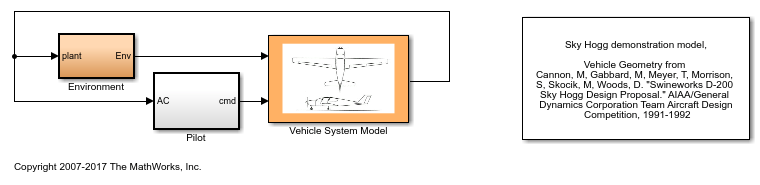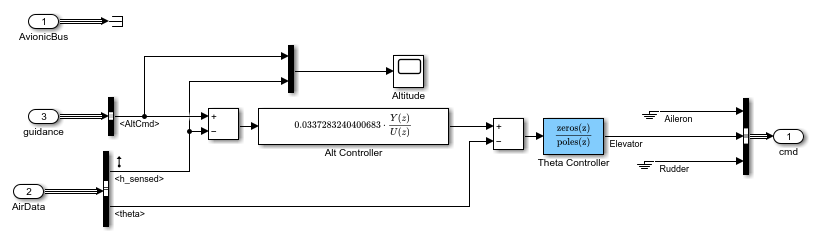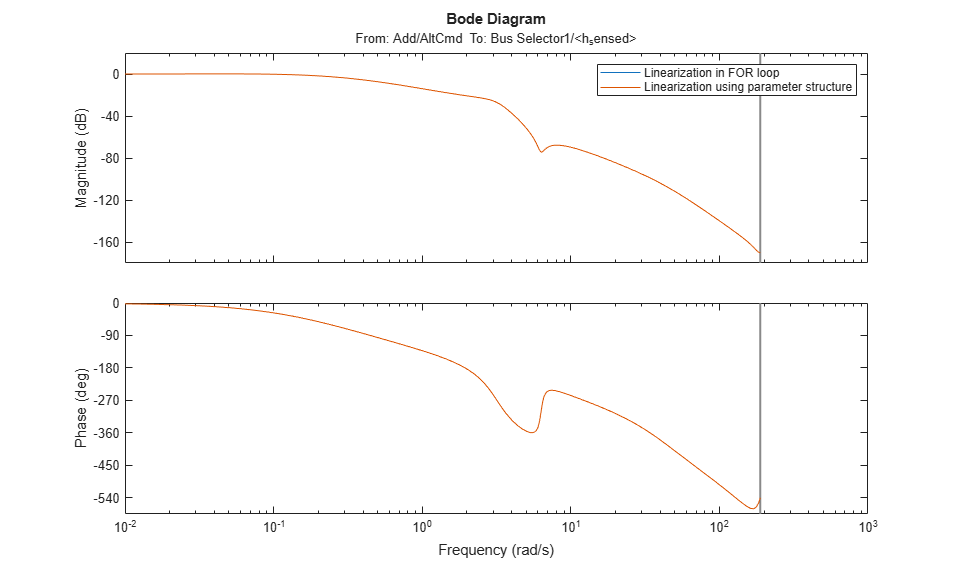# More Efficient Batch Linearization Varying Parameters

This example shows how to speed up the batch linearization of a model when a set of model parameters are varied.

To decrease the linearization time for such a model, you can pass the varying parameter values to the `linearize` function. `linearize` avoids recompiling the model when the varied parameters are tunable parameters. The best improvements in the overall linearization time are for models with large model update times.

### Plant Model

In this example, you linearize a lightweight airplane model. For more information on this model, see Lightweight Airplane Design (Aerospace Blockset). Using this model requires Aerospace Blockset™ software.

Open the model.

```mdl = 'scdskyhogg'; open_system(mdl) ```Extract the linearization inputs and outputs from the model.

```io = getlinio(mdl); ```

Extract the initial operating point of the model.

```op = operpoint(mdl); ```

### Linearize Model By Calling `linearize` Multiple Times

For this example, you vary the gains of the altitude and pitch controllers in the autopilot system by +/- 10%.

Open the auto pilot subsystem.

```open_system('scdskyhogg/Vehicle System Model/Avionics/Autopilot') ```Initialize the gains of the controllers to vary with MATLAB® workspace variables `k1` and `k2`.

```blks = {'scdskyhogg/Vehicle System Model/Avionics/Autopilot/Alt Controller';... 'scdskyhogg/Vehicle System Model/Avionics/Autopilot/Theta Controller'}; set_param(blks{1},'Gain','0.0337283240400683*k1') set_param(blks{2},'Gain','-261.8699347622*k2') ```

Specify the varying values for `k1` and `k2`.

```ct = 1:20; k1val = 1+(ct-10)/100; k2val = 1+(ct-10)/100; ```

Linearize the model for the specified values of `k1` and `k2` by calling the `linearize` function 20 times.

```t = cputime; for i=1:20 k1 = k1val(i); k2 = k2val(i); sys_forloop(:,:,i) = linearize(mdl,op,io); end ```

View the total time in seconds to compute the 20 linearizations.

```dt_for = cputime - t ```
```dt_for = 82.1900 ```

A factor that impacts this time is the total time it takes to compile and evaluate block masks and resolve workspace parameters. To identify bottlenecks in your model compilation, use the MATLAB Profiler.

### Linearize Model By Passing Parameter Values to `linearize`

Instead of calling `linearize` separately for each combination of parameter values, you can pass the parameter values to `linearize` in a single function call.

Define the parameter values in a structure by specifying the name of the MATLAB workspace variables and the value arrays.

```params(1).Name = 'k1'; params(1).Value = k1val; params(2).Name = 'k2'; params(2).Value = k2val; ```

Linearize the model.

```t = cputime; sys_params = linearize(mdl,op,io,params); ```

View the total time to compute the 20 linearizations with one call to the `linearize` function. In this case, the software compiles the model once when varying the specified parameters.

```dt_params = cputime - t ```
```dt_params = 16.6000 ```

### Compare Results

In this example, the varying parameters do not impact the operating point of the Simulink model. The linearizations using both approaches are equivalent.

```bode(sys_forloop(:,:,1),sys_params(:,:,1)) legend('Linearization in FOR loop','Linearization using parameter structure') ```Calculate the resulting time improvement ratio.

```ratio = dt_for/dt_params ```
```ratio = 4.9512 ```

Close the model.

```bdclose(mdl) ```